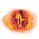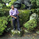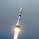5796 views
Hello everyone,

Quick script to check the PEG Ratio.

What is PEG Ratio?
The price/ earnings to growth ratio (PEG Ratio) is a stock's price-to-earnings (P/E) ratio divided by the growth rate of its earnings for a specified time period. The PEG ratio is used to determine a stock's value while also factoring in the company's expected earnings growth, and is thought to provide a more complete picture than the more standard P/E ratio .

A PEG Ratio greater than 1.0 means that the stock is overvalued, while below 1.0 means is is undervalued. When the PEG Ratio is exactly 1.0, then the stock is trading at fair valuation.

Formula:
PEG Ratio = (Price / EPS ) / EPS Growth

Examples:

Company A:
• Price per share = \$46
• EPS this year = \$2.09
• EPS last year = \$1.74
Company B
• Price per share = \$80
• EPS this year = \$2.67
• EPS last year = \$1.78

Company A
• P/E ratio = \$46 / \$2.09 = 22
• Earnings growth rate = (\$2.09 / \$1.74) - 1 = 20%
• PEG ratio = 22 / 20 = 1.1
Company B
• P/E ratio = \$80 / \$2.67 = 30
• Earnings growth rate = (\$2.67 / \$1.78) - 1 = 50%
• PEG ratio = 30 / 50 = 0.6

The company A is overvalued whiled the B is undervalued.

In this script an overvalued stock is considered when the PEG Ratio is above 1.1, while it is 0.9 for an undervalued stock.

Only works with Stocks.

Open-source script

In true TradingView spirit, the author of this script has published it open-source, so traders can understand and verify it. Cheers to the author! You may use it for free, but reuse of this code in a publication is governed by House Rules. You can favorite it to use it on a chart.

Want to use this script on a chart?Do you know of a way to calculate this "manually"? Price is a bar-by-bar change so presumably this can be calculated on a bar-by-bar basis (not just through FQ/FY financials).